# Kerala Syllabus 9th Standard Chemistry Solutions Chapter 1 Structure of Atom

You can Download Structure of Atom Questions and Answers, Summary, Activity, Notes, Kerala Syllabus 9th Standard Chemistry Solutions Part 1 Chapter 1 help you to revise complete Syllabus and score more marks in your examinations.

## Kerala State Syllabus 9th Standard Chemistry Solutions Chapter 1 Structure of Atom

### Structure of Atom Textual Questions and Answers

Kerala Syllabus 9th Standard Chemistry Chapter 1 Question 1.
Explain the postulates of Rutherford model of atom?

• An atom has a center called nucleus
• Compared to the size of an atom the size of the nucleus is very smell
• All the positively charged particles and most of the mass are concentrated in the nucleus of atoms.
• Negatively charged electrons revolve around the nucleus in circular path.
• This atom model is known as planetary model of atom because sun at the center is compared to the nucleus planets revolving around the sun is compared to electrons.

Kerala Syllabus 9th Standard Chemistry Notes Question 2.
Explain the limitations of Rutherford’s model of atom?
According to James Clerk Maxwell’s electromagnetic theory, a charged body in circular motion continuously emits energy as radiations. Electrons which revolve around the nucleus are negatively charged. Hence while electrons revolve around the nucleus, they should emit electromagnetic radiations lose energy and come closer to the nucleus Finally the negatively charged electrons should collapse into the nucleus. But this does not happen in an atom. It Rutherford’s model failed to explain this.

9th Chemistry Notes Kerala Syllabus Question 3.
Explain Bohr model of atoms?

• Electrons revolve around the nucleus in fixed paths called orbit or shells.
• Electrons in each shell have a definite energy hence shells are also called energy levels.
• As long as an electron revolves in a particular orbit its energy remains constant.
• The energy of the shell increases as the distance form the nucleus increases
• The shells.around the nucleus can be numbered from near the nucleus 1,2, 3,4…. or represented by the letters K, L, M, N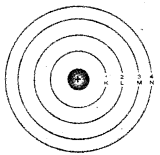Kerala Syllabus 9th Standard Chemistry Guide Pdf Question 4.
Explain the discovery of chargeless particles too- within an atom?
The actual mass of an atom should be equal to the sum of the mass of particles present in it. Scientists realized the fact that there is no relation between the total mass of particle, ie., electrons, and protons in an atom and the actual mass of an atom.

From this, it can be inferred that nucleus may contain neutral particles having mass equal to that of protons predicted by Rutherford. In 1932 James Chad Wick-confirmed the presence of neutral particles within the nucleus of an atom. These particles are called neutrons. The mass of neutron is determined slightly higher than that of protons.

Fundamental particles of an atom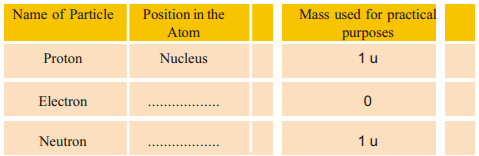Mass Number and Atomic Number

Kerala Syllabus 9th Standard Notes Chemistry Question 5.
Which are the fundamental particles whose masses are mainly responsible for the mass of an atom?
Protons and neutrons

Kerala Syllabus 9th Standard Chemistry Solutions Question 6.
Is there any relation between the total number of protons and neutrons in an atom and its mass?
Same

Chemistry Notes Class 9 Kerala Syllabus Question 7.
Define mass n u m ber of an atom?
The total number of protons and neutrons in an atom is called the mass number it is represented by the Letter ‘A’.

Kerala Syllabus 9th Standard Chemistry Notes Pdf Question 8.
Which is the particle of an atom- that has the possibility of changing of position, which can be exchanged when atoms rub against each other collide or chemically react with other atoms?
Electrons

9th Class Chemistry Notes Kerala Syllabus Question 9.
Atoms are electrically Neutral” Justify it?
The number of protons and electrons are equal in an atom.

Chemistry 9th Class Notes Kerala Syllabus Question 10.
Define atomic number of an element?
The total number of protons in an atom is called atomic number it is represented as Z.

Chemistry Notes For Class 9 Kerala Syllabus Question 11.
Which are the particles whose numbers you can find out if you know the atomic number of an atom?
No. of protons and electrons

Kerala Syllabus 9th Standard Chemistry Notes English Medium Question 12.
Why?
Because the number of protons and electrons in an atom are equal.

Hsslive Guru Chemistry Class 9 Kerala Syllabus Question 13.
How to write the mass number and atomic number of an element if the symbols are known?
Mass number on the left side above the symbol and atomic number on the left side below the symbol.
Eg. Sodium atom can be represented as $$\begin{array}{l}{23 \mathrm{Na}} \\ {\text { 11 }}\end{array}$$

Question 14.
Symbol of certain atoms are given in the table complete the table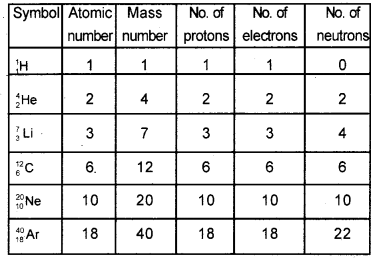Electronic Configuration in Atom

Question 15.
Analyze the electronic configuration of atoms of element 1 – 18 in the following table: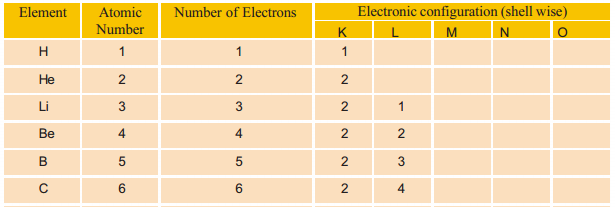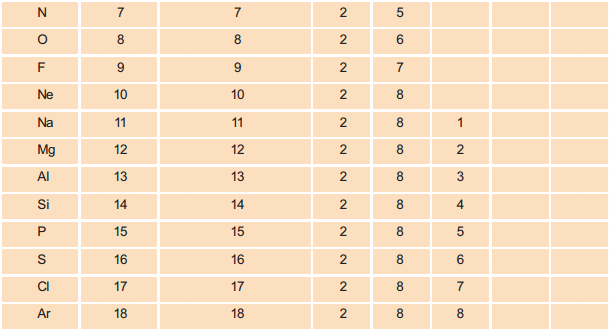Question 16.
What is maximum electrons that can be accommodated in K shell?
2

Question 17.
What is maximum electrons in that can be accommodated in L shell?
8

Rules for filling electrons in shells
1. The maximum electrons that can be accommodated in any given shell can be calculated by the equation 2n2 where ‘n’ represents the number of shells.2. Shells with lower energy will be filled with maximum number of electrons first. Thereafter shells having higher energy will get filled.
3. The maximum number of electrons that can be contained the outermost shell of an atom is 8.

Question 19.
Write down the electron configuration of the following elements Draw its Bohr model.1. $$\frac{14}{7} \mathrm{N}$$ Atomic No.: -7
Electron configuration – 2, 5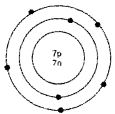2. $$\frac{24}{12} \mathrm{Mg}$$ Atomic No.: 12
Electron configuration – 2, 8,2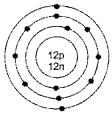3. $$\frac{32}{16} \mathrm{S}$$ Atomic No.: -16
Electron configuration – 2, 8,6Question 20.
Bohr model of atoms of certain elements are given below (Fig 1.9) asess their electronic configuration.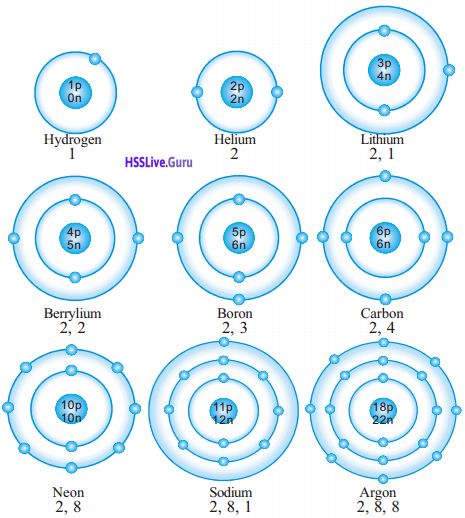Question 21.
The symbol of the aluminum atom is $$\frac{27}{13} \mathrm{AI}$$ Bohr model of the atom is given in Fig. 1.10 analyze these and complete the table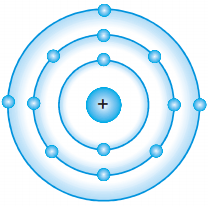Atomic number 13 Mass number 27 Number of Protons 13 Number of electrons 13 Number of neutrons 14 Electron configuration 2, 8, 3

Isotopes

Question 22.
You would have understood that the number of protons in an atom determines the element. Analyze the given Bohr models.Question 23.
Complete the table providing details related to these atoms.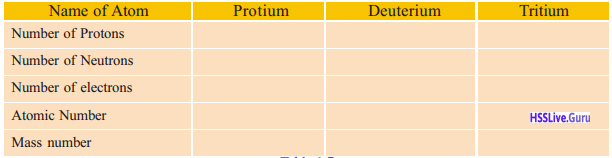Name of atom Protium Deuterium Tritium Number of protons 1 1 1 Number of neutrons 0 1 2 Number of electrons 1 1 1 Atomic number 1 1 1 Mass number 1 2 3

Question 24.
Among these atoms which is the particle that is differs in numbers?
Neutrons

Question 25.
Which inferences can you arrive at when you examine the atomic number and mass number of these elements?
They are atoms of the same element with same atomic number. But different mass numbers.

Question 26.
What is meant by Isotopes?
Isotopes are atoms of the same element with same atomic number but different mass number.

Question 27.
Which isotope of Hydrogen is used in atomic reactors?
Deuterium

Question 28.
Write the isotopes of carbon?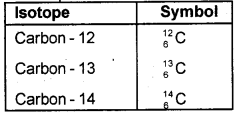Question 29.
Which are the isotopes is used as tracers for identifying the nutrient exchange in plant?
Phosphorous-31

Question 30.
Which isotope of Uranium is used in atomic reac¬tors as fuel?
Uranium – 235

Question 31.
What is meant by isobar?
Isobars are atoms having the same mass number but different atomic number
e.g. $$_{20}^{40} \mathrm{Ca}, \quad_{18}^{40} \mathrm{Ar}$$

Question 32.
Symbols of certain isotopes are given in table 1.9. Complete the table writing their atomic number, mass number, number of protons, electrons and neutrons.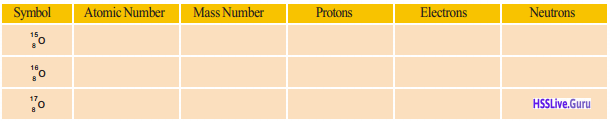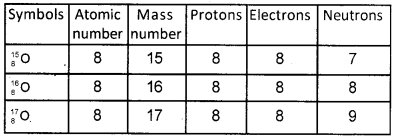Let Us Assess

Question 1.
Name of some scientists and their contributions are given in in the following table. Match them suitably in the chronological order.

 Scientist Contribution John Dalton Law of Electrolysis Michael Faraday Planetary Model of Atom J.J. Thomson Atomic theory Rutherford Discovered electron

 Scientist Contribution John Dalton Atomic theory Michael Faraday Law of Electrolysis J.J. Thomson Discovered electron Rutherford Planetary Model of Atom

Question 2.
Atomic number of an atom Z=17, Mass number 35.
a) Find the number of protons, electrons, and neutrons in the atom.
b) Write the electronic configuration of different shells.
c) Draw the Bohr model of atom.
a) No. of protons -17
No. of electrons -17 No. of neutrons = 35 – 17 =18
b) 2, 8, 7Question 3.
The mass number of an atom is 31. The M shell of this atom contains 5 electrons.
a) Write the electronic configuration.
b) What is the atomic number of this atom?
c) How many neutrons does this atom have?
d) Draw the Bohr model of the atom.
a) 2, 8, 5
b) 15
c) 31 – 15 = 16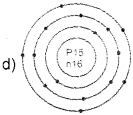Question 4.
Bohr models of atoms A, B, C, D are given (Symbols are not real).
a) Write the atomic number, mass number and electronic configuration of the atoms.
b) Among these, which are isotopes? Why?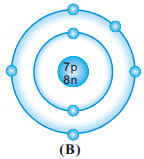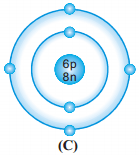Element Atomic Number Mass Number Electron Configuration A 6 12 2,4 B 7 15 2, 8, 5 C 6 14 2,4

Question 5.
Symbols (not real symbols) of some atoms are given.
$$_{17}^{8} \mathrm{P}, \quad_{40}^{18} \mathrm{Q}$$$$_{8}^{16} \mathrm{P}, \quad_{40}^{20} \mathrm{R}$$
a) Find the atomic number and mass number of these elements.
b) Which among these are isotopic pairs?
c) Draw the Bohr model of atom Q
a)
b) Isotopes P, R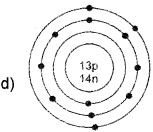### Structure of Atom Model Questions and Answers

Question 1.
Write the atomic number of an atom is 18 and mass number is 40
a) Find the number of protons, electrons, and neutrons in the atom.
b) Write the electronic configuration of different shells
c) Draw the Bohr model of the atom
a) No. of protons -18
No. of electrons -18
No. of neutrons – 40 – 18 = 22
b) 2, 8, 8Question 2.
The 3rd shell ie. M shell of an atom contains 3 electrons. The mass number is 27.
a) Write the atomic number of this atom.
b) Write the electronic configuration.
c) How many electrons, neutrons does this atoms have?
d) Draw the Bohr model of atom?
a) 13
b) 2, 8, 3
c) No. of protons -13 No. of electrons -13
No. of neutrons – 27 – 13 = 14Question 3.
Write the uses of the following isotope
a) Carbon – 14
b) Phosphorous – 31
c) Iodine – 131 and cobalt 60
d) Uranium – 235
Isotope Uses
a) Carbon-14 — Used to determine the age of fossils and prehistoric objects
b) Phosphorous-31 — Used as tracers for identifying the nutrient exchange in plants
c) Iodine -131 or cobalt – 60 — In medical sciences for the diagnosis and treatment of cancer and tumor
d) Uranium – 235 — Used in atomic reactor as fuel

Question 4.
Write the electronic configuration and draw the Bohr model?
1) $$_{35}^{17} \mathrm{CI}$$
Electron configuration : 2, 8, 7
No. of neutrons = 35 – 17 = 18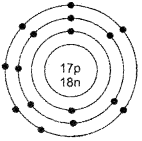2) $$_{23}^{11} \mathrm{Na}$$
No. of neutrons = 23 – 11 = 12
Electron configuration -2,8,1Question 5.
$$\frac{12}{6} \mathrm{C}, \frac{14}{6} \mathrm{C}$$
Find the isotopes and isobars pairs among them. Justify it?
$$\frac{40}{18} \mathrm{Ar}, \frac{40}{20} \mathrm{Ca}$$ are Isotopes. Because they are atoms of the same element with same atomic number but different mass numbers.